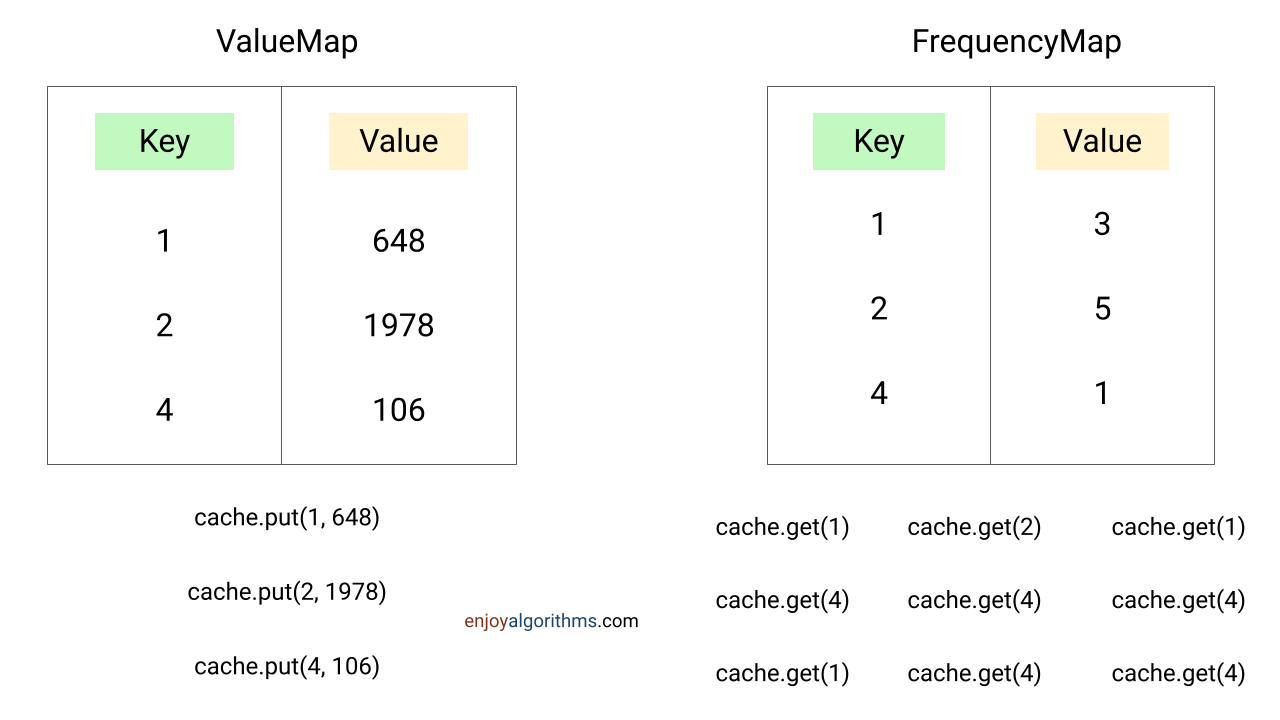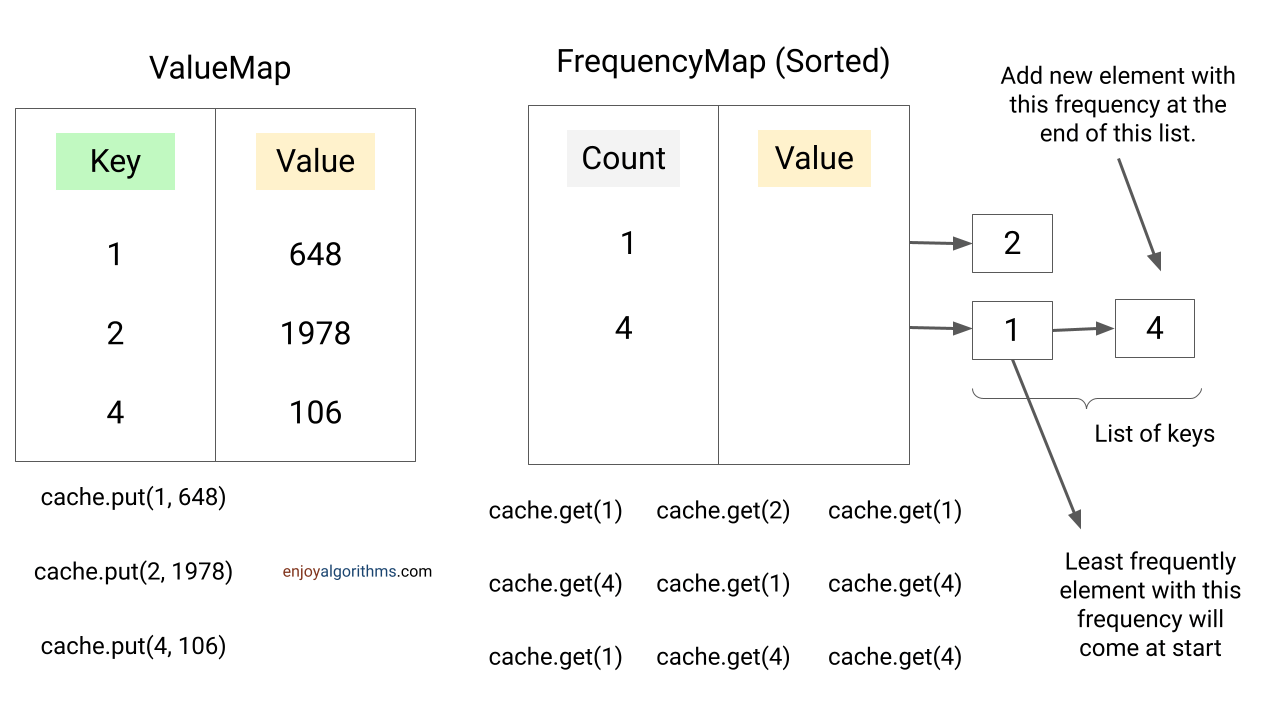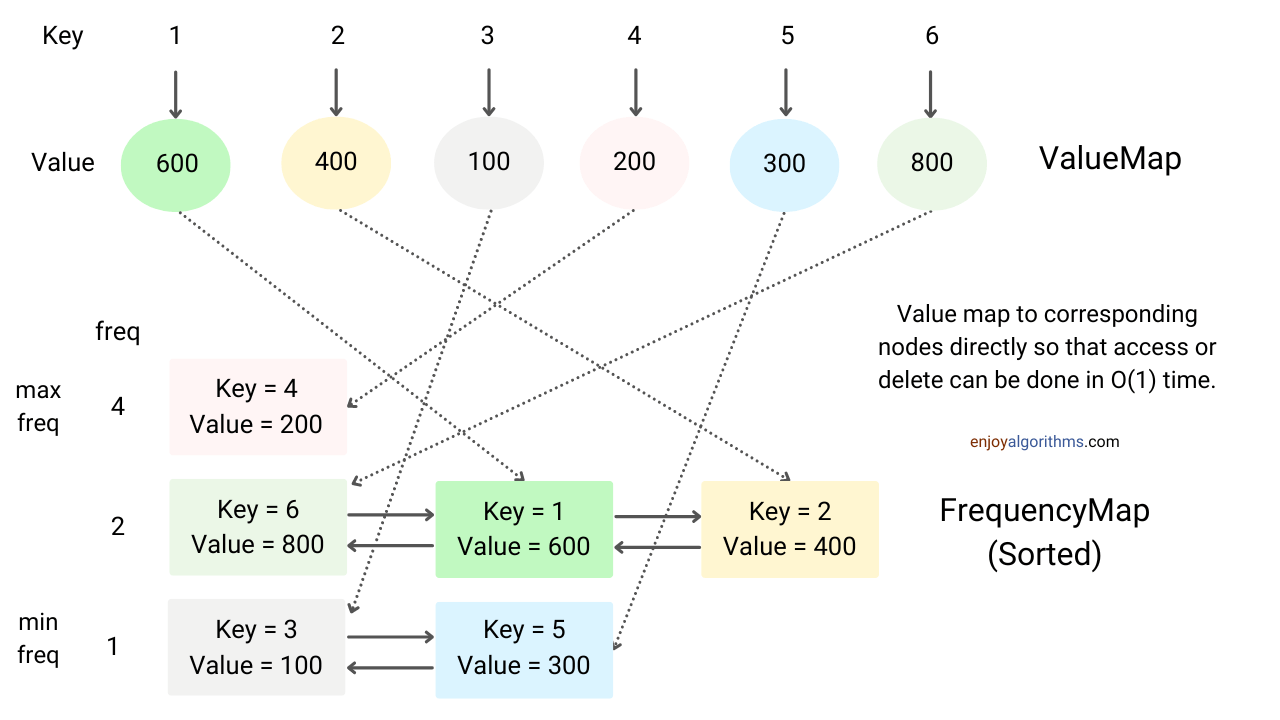# Design and Implement Least Frequently Used (LFU) Cache

### What is LFU Cache?

Least Frequently Used is a cache algorithm used to manage memory within a computer. In this method, system keeps track of number of times a block is referenced in memory. The system removes the item with the lowest reference frequency when the cache is full.

### Problem Statement of LFU Cache

Our goal is to design a data structure that follows the constraints of a Least Frequently Used(LFU) cache. Our LFU cache should support the following operations:

• LFUCache(int capacity): Initialises the object with the capacity of the data structure.
• int get(int key): Returns the value of the key if the key exists in the cache; otherwise, returns -1.
• void put(int key, int value): Update the value of the key if present or insert the key if not already present. When the cache reaches its capacity, it should invalidate and remove the least frequently used key before inserting a new item. If there is a tie, i.e. two or more keys with the same frequency, the least recently used key would be invalidated.

The frequency for a key in the cache is incremented when either a get or put operation is called on it. The operations get and put must each run in O(1) average time complexity.

### Brute force approach to implement LFU Cache

We initialize an array of sizes equal to that of our cache. Each element stores the key and value along with the frequency and the time at which this key is accessed. We use this frequency and the timestamp to determine the least frequently used element.

``````class Element
{
int key;
int val,
int frequency;
int timeStamp

public Element(int k, int v, int time)
{
key = k;
val = v;
frequency = 1;
timeStamp = time;
}

}``````

int get(int key): We traverse the array and compare the key of each element in our cache with the given key. If we find the key of an element equal to the key, we increment the frequency of this element and update the timestamp. If we do not find any element, we return -1. Time complexity = O(n).

void put(int key, int value): If the array is not full, we create a new element with frequency 1 and timestamp 0. We increase the timestamp of the existing data in the array by 1. If the array is full, we must delete the least frequently used element. For this, we iterate through the array and find the element with the least frequency. In the case of frequency ties, we consider the least recently used element (oldest timestamp). Now we insert the new element.

### An efficient approach to implement LFU Cache

First, we will implement the insert and access operations in O(1) time. We need two maps to achieve this, one to store the key-value pairs and the second one to store counts/frequency of access.``````public class LFUCache
{
private Map<Integer, Integer> valueMap = new HashMap<>();
private Map<Integer, Integer> frequencyMap = new HashMap<>();
private final int size;
public LFUCache(int capacity)
{
size = capacity;
}

public int get(int key)
{
if(valueMap.containsKey(key) == false)
return -1;
frequencyMap.put(key, frequencyMap.get(key) + 1);
return valueMap.get(key);

}

public void put(int key, int value)
{
if(valueMap.containsKey(key) == false)
{
valueMap.put(key, value);
frequencyMap.put(key, 1);
}
else
{
valueMap.put(key, value);
frequencyMap.put(key, frequencyMap.get(key) + 1);
}
}
}``````

Now in the above code, we need to implement the code for the eviction strategy. When the map size reaches the max capacity, we need to find the item with the lowest frequency count. In our current implementation, we have to iterate through all the values of the frequencyMap and find the lowest count and remove the corresponding key from both the maps. This takes O(n) time.

Also, if multiple keys have the same frequency count, we cannot find the least recently used key with our current implementation as HashMap does not store the insertion order. To handle this, we add one more data structure, a sorted map with the key as the frequency and value as a list of elements with the same frequency.Now, new items can be added to the end of the list with frequency 1. Since the map stores the frequencies in sorted order, we can find the list with the lowest frequency in O(1) time. Also, we can delete the list’s first item (of lowest frequency) since that will be the least recently used in O(1) time.

### LFU cache implementation in java: Using a singly linked list

``````public class LFUCache
{
private Map<Integer, Integer> valueMap = new HashMap<>();
private Map<Integer, Integer> countMap = new HashMap<>();
private TreeMap<Integer, List<Integer>> frequencyMap = new TreeMap<>();
private final int size;

public LFUCache(int capacity)
{
size = capacity;
}

public int get(int key)
{
if(valueMap.containsKey(key) == false || size == 0)
return -1;
//remove element from current frquency list and move it to the next containsKey
// Not O(1)
int frequency = countMap.get(key);
frequencyMap.get(frequency).remove(new Integer(key));

//remove frquency from map if list is empty
if(frequencyMap.get(frequency).size() == 0)
frequencyMap.remove(frequency);

countMap.put(key, frequency + 1);
return valueMap.get(key);
}

public void put(int key, int value)
{
if(valueMap.containsKey(key) == false && size > 0)
{
if(valueMap.size() == size)
{
// remove first element from the list
//element with minimum frrquency
lowestCount = frequencyMap.firstKey();
int keyToDelete = frequencyMap.get(lowestCount).remove(0);

//remove frquency from map if list is empty
if(frequencyMap.get(lowestCount).size() == 0)
frequencyMap.remove(lowestCount);

valueMap.remove(keyToDelete) ;
countMap.remove(keyToDelete);
}

valueMap.put(key, value);
countMap.put(key, 1);

}
else if(size > 0)
{
//update value
valueMap.put(key, value);
// update count and frequency map
int frequency = countMap.get(key);
frequencyMap.get(frequency).remove(new Integer(key));

//remove frquency from map if list is empty
if(frequencyMap.get(frequency).size() == 0)
frequencyMap.remove(frequency);

countMap.put(key, frequency + 1);
}
}
}``````

Thus, both insert and delete operations are O(1), i.e. constant-time operations.

While solving the eviction problem, we have increased the access operation time to O(n). All of the item-keys sharing the same frequency are in a list. Now, if one of these items is accessed, we need to move it to the list of the following frequency. We will have to iterate through the list first to find the item, which in worst-case will take O(n) operations.

We somehow need to access that item directly in the list without iteration to solve this problem. If we can do this, we can delete this item in O(1) time from the current frequency list and move it at the end of the list of the following frequency list in O(1) time.

For this, we need a doubly linked list. We will create a node that stores an item’s key, value, and position in the list. We will convert the list to a doubly-linked list.### LFU Cache Implementation Using Doubly Linked List (Java)

``````public class LFUCache {

private Map<Integer, Node> valueMap = new HashMap<>();
private Map<Integer, Integer> countMap = new HashMap<>();
private TreeMap<Integer, DoubleLinkedList> frequencyMap = new TreeMap<>();
private final int size;

public LFUCache(int n) {
size = n;
}

public int get(int key) {
if (!valueMap.containsKey(key) || size == 0) {
return -1;
}

// Remove element from current frequency list and move it to the next one in O(1) time
Node nodeTodelete = valueMap.get(key);
Node node = new Node(key, nodeTodelete.value());
int frequency = countMap.get(key);
frequencyMap.get(frequency).remove(nodeTodelete);
removeIfListEmpty(frequency);
valueMap.remove(key);
countMap.remove(key);
valueMap.put(key, node);
countMap.put(key, frequency + 1);
return valueMap.get(key).value;
}

public void put(int key, int value) {
if (!valueMap.containsKey(key) && size > 0){

Node node = new Node(key, value);

if (valueMap.size() == size) {
// remove the first node(LRU) from the list the of smallest frequency(LFU)
int lowestCount = frequencyMap.firstKey();
frequencyMap.get(lowestCount).remove(nodeTodelete);
removeIfListEmpty(lowestCount);

int keyToDelete = nodeTodelete.key();
valueMap.remove(keyToDelete);
countMap.remove(keyToDelete);
}
valueMap.put(key, node);
countMap.put(key, 1);

}
else if(size > 0){
Node node = new Node(key, value);
Node nodeTodelete = valueMap.get(key);
int frequency = countMap.get(key);
frequencyMap.get(frequency).remove(nodeTodelete);
removeIfListEmpty(frequency);
valueMap.remove(key);
countMap.remove(key);
valueMap.put(key, node);
countMap.put(key, frequency + 1);

}
}

private void removeIfListEmpty(int frequency) {
// remove from map if list is empty
if (frequencyMap.get(frequency).len() == 0) {
frequencyMap.remove(frequency);
}
}

private class Node {
private int key;
private int value;
Node next;
Node prev;

public Node(int key, int value) {
this.key = key;
this.value = value;
}

public int key() {
return key;
}

public int value() {
return value;
}
}

private int n;
private Node tail;

} else {
tail.next = node;
node.prev = tail;
}
tail = node;
n++;
}

public void remove(Node node) {

if (node.next == null) tail = node.prev;
else node.next.prev = node.prev;

if (node.prev == null) head = node.next;
else node.prev.next = node.next;

n--;
}

}

public int len() {
return n;
}
}

}``````

### Real-World Application of LFU Cache

Consider a caching network proxy application for the HTTP protocol. This proxy typically sits between the internet and the user or a set of users. It ensures that all the users can access the internet and enables sharing of all the shareable resources for optimum network utilisation and improved responsiveness. Such a caching proxy should maximize the amount of data it can cache in the limited amount of storage or memory at its disposal. Typically, many static resources such as images, CSS style sheets, and javascript code can quickly be cached for a reasonably long time before newer versions replace them. These static resources are included in pretty much every page, so it is most beneficial to cache them since pretty much every request will require them. Furthermore, since a network proxy must serve thousands of requests per second, the overhead needed to do so should be kept to a minimum.

It should evict only those resources that are not used very frequently to that effect. Hence, the frequently used resources should be kept at the expense of the not so frequently used ones since the former has proved helpful over a while. Thus, these caching proxies can employ the LFU cache replacement strategy to evict the least frequently used items in its cache when there is a lack of memory. LRU cache might also be an appropriate strategy here, but it would fail when the request pattern is such that all requested items do not fit into the cache and the items are requested in a round-robin fashion. In the case of LRU, items will constantly enter and leave the cache, with no user request ever hitting the cache. Under the same conditions, however, the LFU algorithm will perform much better, with most cached items resulting in a cache hit.

Enjoy learning, Enjoy Algorithms!

☆ 16-Week Live DSA Course
☆ 10-Week Live DSA Course